# Explore the mesh#

This example shows how to explore and manipulate the mesh object to query mesh data such as connectivity tables, element IDs, element types and so on.

## Perform required imports#

Perform required imports. This example uses a supplied file that you can get by importing the DPF `examples` package.

```from ansys.dpf import post
from ansys.dpf.post import examples
from ansys.dpf.post.common import elemental_properties
```

Load the result file in a `Simulation` object that allows access to the results. The `Simulation` object must be instantiated with the path for the result file. For example, `"C:/Users/user/my_result.rst"` on Windows or `"/home/user/my_result.rst"` on Linux.

```example_path = examples.download_harmonic_clamped_pipe()
simulation = post.HarmonicMechanicalSimulation(example_path)
```

## Get the mesh#

Retrieve the mesh and print it

```mesh = simulation.mesh
print(mesh)
```
```DPF  Mesh:
9943 nodes
5732 elements
Unit: mm
With solid (3D) elements, shell (2D) elements, shell (3D) elements
```

## Plot the mesh#

Plot the mesh to view the bare mesh of the model

```mesh.plot()
```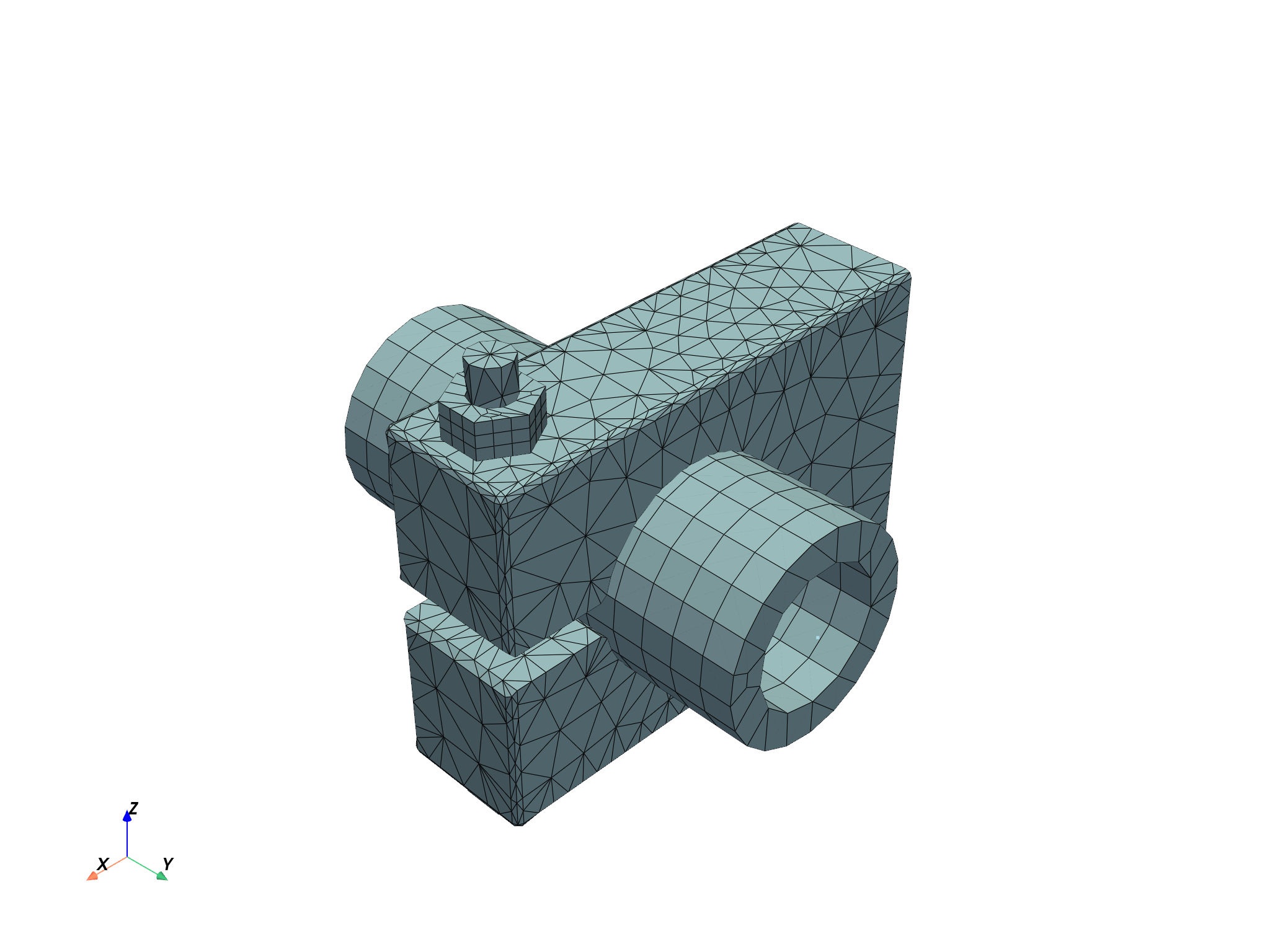## Query basic information about the mesh#

The `Mesh` object has several properties allowing access to different information such as:

the number of nodes

```print(f"This mesh contains {mesh.num_nodes} nodes")
```
```This mesh contains 9943 nodes
```

the list of node IDs

```print(f"with IDs: {mesh.node_ids}")
```
```with IDs: [   1    2    3 ... 9941 9942 9943]
```

the number of elements

```print(f"This mesh contains {mesh.num_elements} elements")
```
```This mesh contains 5732 elements
```

the list of element IDs

```print(f"with IDs {mesh.element_ids}")
```
```with IDs [3487 3960 1449 ... 8438 8437 8540]
```

the unit of the mesh

```print(f"The mesh is in '{mesh.unit}'")
```
```The mesh is in 'mm'
```

## Named selections#

The available named selections are given as a dictionary with the names as keys and the actual `NamedSelection` objects as values. Printing the dictionary informs you on the available names.

```named_selections = mesh.named_selections
print(named_selections)
```
```NamedSelections dictionary with 4 named selections:
- 'CLAMP'
- 'PIPE'
- 'SCREW'
- '_FIXEDSU'
```

To get a specific named selection, query it using its name as key

```print(named_selections["_FIXEDSU"])
```
```NamedSelection '_FIXEDSU'
with DPF  Scoping:
with Nodal location and 161 entities
```

## Elements#

Use `mesh.elements` to access the list of Element objects

```print(mesh.elements)
```
```[tet10, ..., point1]
```

You can then query a specific element by its ID

```print(mesh.elements.by_id)
```
```DPF Element 1
Index:         4239
Nodes:           20
Type:         Hex20
Shape:        Solid
```

or by its index

```element_0 = mesh.elements
print(element_0)
```
```DPF Element 3487
Index:            0
Nodes:           10
Type:         Tet10
Shape:        Solid
```

## Query information about a particular element#

You can request the IDs of the nodes attached to an `Element` object

```print(element_0.node_ids)
```
```[3548, 3656, 4099, 3760, 6082, 6650, 6086, 6085, 6647, 7147]
```

or the list of `Node` objects

```print(element_0.nodes)
```
```[<ansys.dpf.core.nodes.Node object at 0x7ff39042cee0>, <ansys.dpf.core.nodes.Node object at 0x7ff39042c850>, <ansys.dpf.core.nodes.Node object at 0x7ff39042c640>, <ansys.dpf.core.nodes.Node object at 0x7ff39042cc40>, <ansys.dpf.core.nodes.Node object at 0x7ff39042ce20>, <ansys.dpf.core.nodes.Node object at 0x7ff39042c7f0>, <ansys.dpf.core.nodes.Node object at 0x7ff39042cc70>, <ansys.dpf.core.nodes.Node object at 0x7ff39042c340>, <ansys.dpf.core.nodes.Node object at 0x7ff39042c4c0>, <ansys.dpf.core.nodes.Node object at 0x7ff3b4cf9cd0>]
```

To get the number of nodes attached, use

```print(element_0.num_nodes)
```
```10
```

Get the type of the element

```print(element_0.type_info)
print(element_0.type)
```
```Element Type
------------
Enum id (dpf.element_types): element_types.Tet10
Element name (short): tet10
Element shape: solid
Number of corner nodes: 4
Number of mid-side nodes: 6
Total number of nodes: 10
element_types.Tet10
```

Get the shape of the element

```print(element_0.shape)
```
```solid
```

## Element types and materials#

The `Mesh` object provides access to properties defined on all elements, such as their types or their associated materials.

Get the type of all elements

```print(mesh.element_types)
```
```    results elem_type_id
element_ids
3487            0
3960            0
1449            0
3131            0
3124            0
3126            0
...          ...
```

Get the materials of all elements

```print(mesh.materials)
```
```    results material_id
element_ids
3487           1
3960           1
1449           1
3131           1
3124           1
3126           1
...         ...
```

## Elemental connectivity#

The elemental connectivity maps elements to connected nodes, either using IDs or indexes.

To access the indexes of the connected nodes using an element’s index, use

```element_to_node_connectivity = mesh.element_to_node_connectivity
print(element_to_node_connectivity)
```
```[3547, 3655, 4098, 3759, 6081, 6649, 6085, 6084, 6646, 7146]
```

To access the IDs of the connected nodes using an element’s index, use

```element_to_node_ids_connectivity = mesh.element_to_node_ids_connectivity
print(element_to_node_ids_connectivity)
```
```[3548, 3656, 4099, 3760, 6082, 6650, 6086, 6085, 6647, 7147]
```

Each connectivity object has a `by_id` property which changes the input from index to ID, thus:

To access the indexes of the connected nodes using an element’s ID, use

```element_to_node_connectivity_by_id = mesh.element_to_node_connectivity.by_id
print(element_to_node_connectivity_by_id)
```
```[3547, 3655, 4098, 3759, 6081, 6649, 6085, 6084, 6646, 7146]
```

To access the IDs of the connected nodes using an element’s ID, use

```element_to_node_ids_connectivity_by_id = mesh.element_to_node_ids_connectivity.by_id
print(element_to_node_ids_connectivity_by_id)
```
```[3548, 3656, 4099, 3760, 6082, 6650, 6086, 6085, 6647, 7147]
```

## Nodes#

Get a node by its ID

```node_1 = mesh.nodes.by_id
print(node_1)
```
```Node(id=1, coordinates=[44.90718016, 12.57776697, 53.33333333])
```

Get a node by its index

```print(mesh.nodes)
```
```Node(id=1, coordinates=[44.90718016, 12.57776697, 53.33333333])
```

Get the coordinates of all nodes

```print(mesh.coordinates)
```
```            results  coord (m)
node_ids components
1          X 4.4907e+01
Y 1.2578e+01
Z 5.3333e+01
2          X 4.4907e+01
Y 1.2578e+01
Z 5.1667e+01
...        ...        ...
```

## Query information about one particular node#

Get the coordinates of a node

```print(node_1.coordinates)
```
```[44.90718016, 12.57776697, 53.33333333]
```

## Nodal connectivity#

The nodal connectivity maps nodes to connected elements, either using IDs or indexes.

To access the indexes of the connected elements using a node’s index, use

```node_to_element_connectivity = mesh.node_to_element_connectivity
print(node_to_element_connectivity)
```
```[4216, 4218, 4219, 4242, 4244, 4245]
```

To access the IDs of the connected elements using a node’s index, use

```node_to_element_ids_connectivity = mesh.node_to_element_ids_connectivity
print(node_to_element_ids_connectivity)
```
```[11, 8, 14, 10, 7, 13]
```

Each connectivity object has a `by_id` property which changes the input from index to ID, thus:

To access the indexes of the connected elements using a node’s ID, use

```node_to_element_connectivity_by_id = mesh.node_to_element_connectivity.by_id
print(node_to_element_connectivity_by_id)
```
```[4216, 4218, 4219, 4242, 4244, 4245]
```

To access the IDs of the connected elements using a node’s ID, use

```node_to_element_ids_connectivity_by_id = mesh.node_to_element_ids_connectivity.by_id
print(node_to_element_ids_connectivity_by_id)
```
```[11, 8, 14, 10, 7, 13]
```

## Splitting into meshes#

You can split the global mesh according to mesh properties to work on specific parts of the mesh

```meshes = simulation.split_mesh_by_properties(
properties=[elemental_properties.material, elemental_properties.element_shape]
)
```

The object obtained is a `Meshes`

```print(meshes)
```
```DPF Meshes Container with
14 mesh(es)
defined on labels ['elshape', 'mat']
```

Plotting a `Meshes` object plots a combination of all the `Mesh` objects within

```meshes.plot(text="Mesh split")
```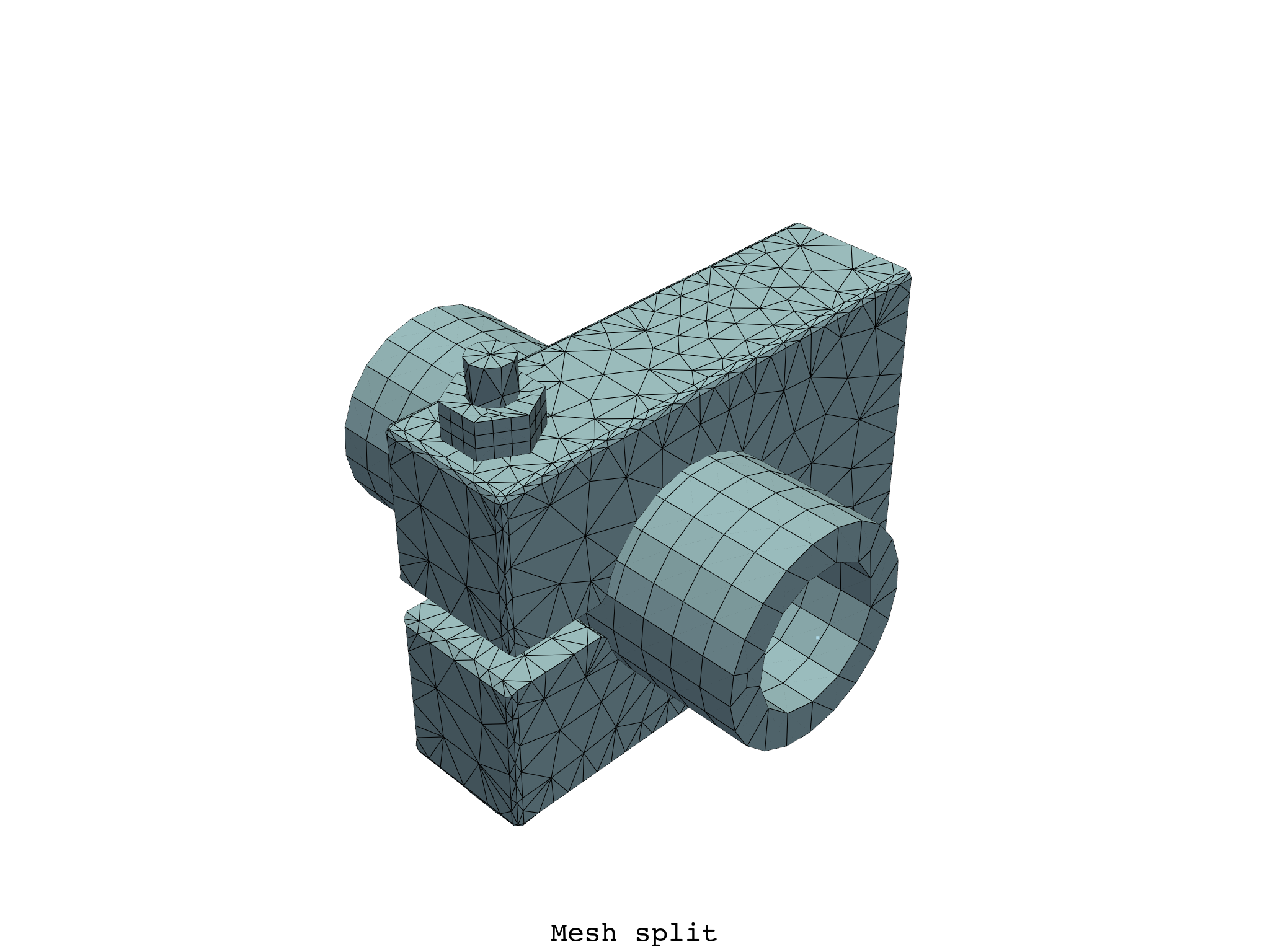Select a specific `Mesh` in the `Meshes`, by index

```meshes.plot(text="First mesh in the split mesh")
```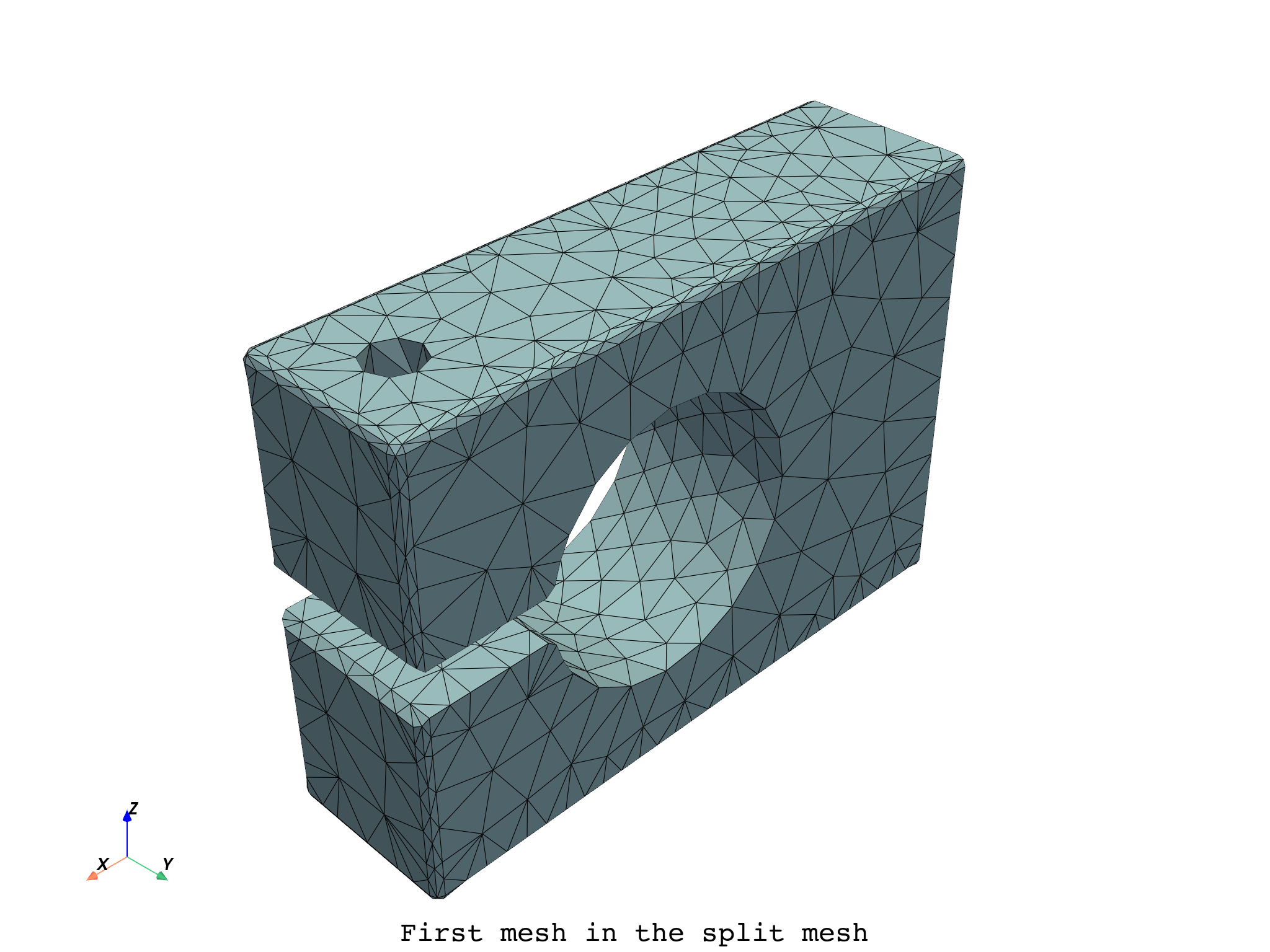You can split the global mesh and select meshes based on specific property values

```meshes_filtered = simulation.split_mesh_by_properties(
properties={
elemental_properties.material: [2, 3, 4],
elemental_properties.element_shape: 1,
}
)
meshes_filtered.plot(text="Mesh split and filtered")
```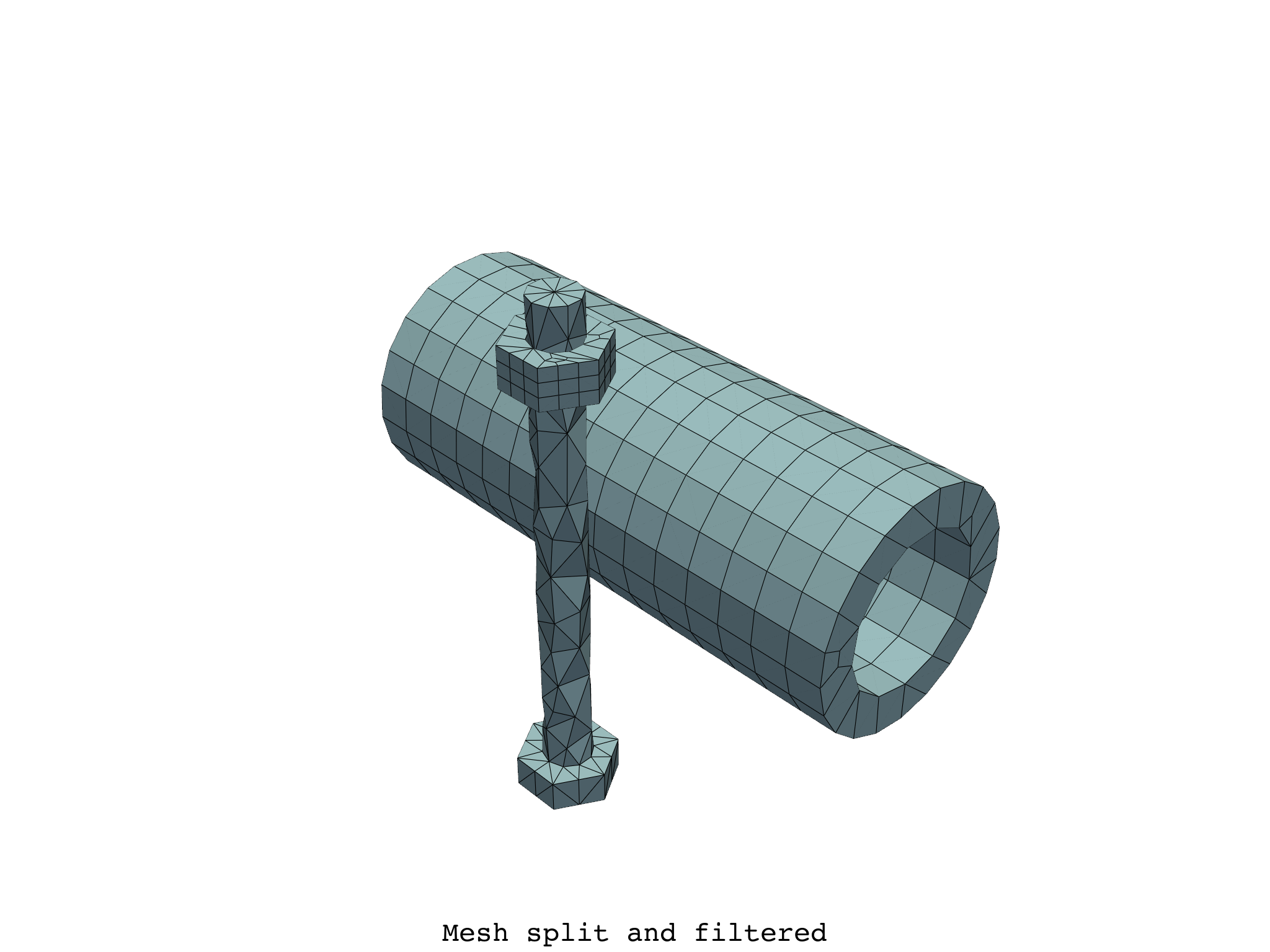or with a unique combination of property values

```meshes[{"mat": 5, "elshape": 0}].plot(text="Mesh for mat=5 and elshape=0")
```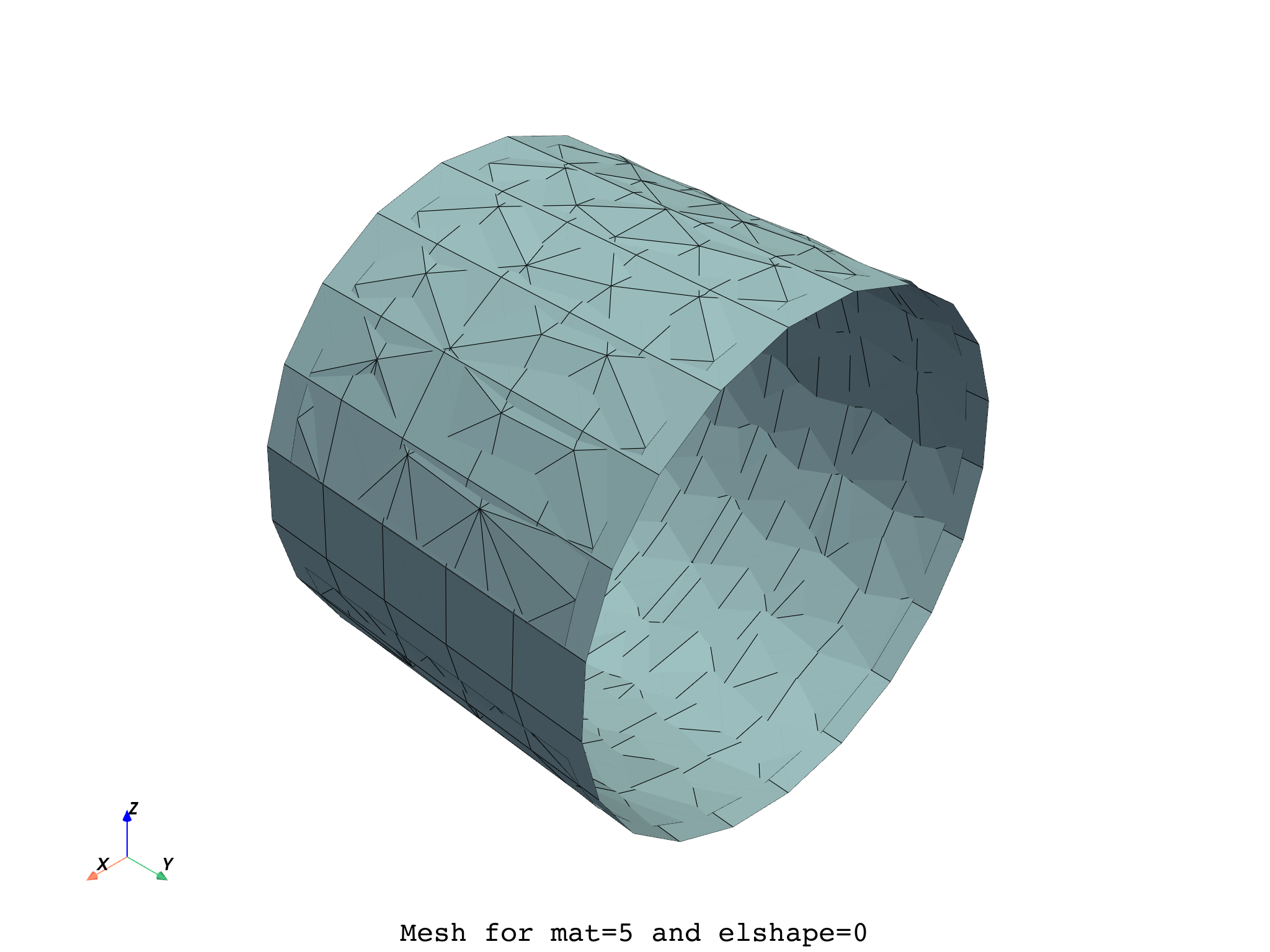Total running time of the script: ( 0 minutes 16.549 seconds)

Gallery generated by Sphinx-Gallery